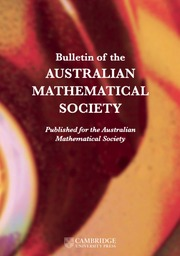Home
Hostname: page-component-78bd46657c-jcldq Total loading time: 0.274 Render date: 2021-05-06T17:09:25.667Z Has data issue: true Feature Flags: { "shouldUseShareProductTool": true, "shouldUseHypothesis": true, "isUnsiloEnabled": true, "metricsAbstractViews": false, "figures": false, "newCiteModal": false, "newCitedByModal": true }Bulletin of the Australian Mathematical Society

# ON PROBLEMS OF$\boldsymbol{\mathcal{CF}}$-CONNECTED GRAPHS FOR$\boldsymbol{K}_{\boldsymbol{m,n}}$

Published online by Cambridge University Press:  01 December 2020

## Abstract

A connected graph G is$\mathcal {CF}$ -connected if there is a path between every pair of vertices with no crossing on its edges for each optimal drawing of G. We conjecture that a complete bipartite graph$K_{m,n}$ is$\mathcal {CF}$ -connected if and only if it does not contain a subgraph of$K_{3,6}$ or$K_{4,4}$ . We establish the validity of this conjecture for all complete bipartite graphs$K_{m,n}$ for any$m,n$ with$\min \{m,n\}\leq 6$ , and conditionally for$m,n\geq 7$ on the assumption of Zarankiewicz’s conjecture that$\mathrm {cr}(K_{m,n})=\big \lfloor \frac {m}{2} \big \rfloor \big \lfloor \frac {m-1}{2} \big \rfloor \big \lfloor \frac {n}{2} \big \rfloor \big \lfloor \frac {n-1}{2} \big \rfloor$ .

## MSC classification

Type
Research Article
© 2020 Australian Mathematical Publishing Association Inc.

## Access options

Get access to the full version of this content by using one of the access options below.

## References

Bokal, D. and Leaños, J., ‘Characterizing all graphs with$2$ -exceptional edges’, Ars Math. Contemp. 15(2) (2018), 383406.CrossRefGoogle Scholar
Cairns, G., Mendan, S. and Nikolayevsky, Y., ‘A sufficient condition for a pair of sequences to be bipartite graphic’, Bull. Aust. Math. Soc. 94(2) (2016), 195200.CrossRefGoogle Scholar
Christian, R., Richter, R. B. and Salazar, G., ‘Zarankiewicz’s conjecture is finite for each fixed$m$ ’, J. Combin. Theory Ser. B 103(2) (2013), 237247.CrossRefGoogle Scholar
Guy, R. K., ‘The decline and fall of Zarankiewicz’s theorem’, in: Proof Techniques in Graph Theory (ed. Harary, F.) (Academic Press, New York, 1969), 6369.Google Scholar
Kleitman, D. J., ‘The crossing number of${K}_{5,n}$ ’, J. Combin. Theory 9 (1970), 315323.CrossRefGoogle Scholar
Klešč, M., ‘The crossing number of join of the special graph on six vertices with path and cycle’, Discrete Math. 310(9) (2010), 14751481.CrossRefGoogle Scholar
Norin, S. and Zwols, Y., ‘Turan’s brickyard problem and flag algebras’, BIRS Workshop on Geometric and Topological Graph Theory, 2013 (presentation 13w5091), available online at http://www.birs.ca/events/2013/5-day-workshops/13w5091/videos/watch/201310011538-Norin.html.Google Scholar
Woodall, D. R., ‘Cyclic-order graphs and Zarankiewicz’s crossing number conjecture’, J. Graph Theory 17(6) (1993), 657671.CrossRefGoogle Scholar
Zarankiewicz, K., ‘On a problem of P. Turan concerning graphs’, Fund. Math. 41(1) (1955), 137145.CrossRefGoogle Scholar

# Send article to Kindle

Note you can select to send to either the @free.kindle.com or @kindle.com variations. ‘@free.kindle.com’ emails are free but can only be sent to your device when it is connected to wi-fi. ‘@kindle.com’ emails can be delivered even when you are not connected to wi-fi, but note that service fees apply.

Find out more about the Kindle Personal Document Service.

ON PROBLEMS OF$\boldsymbol{\mathcal{CF}}$-CONNECTED GRAPHS FOR$\boldsymbol{K}_{\boldsymbol{m,n}}$
Available formats
×

# Send article to Dropbox

To send this article to your Dropbox account, please select one or more formats and confirm that you agree to abide by our usage policies. If this is the first time you use this feature, you will be asked to authorise Cambridge Core to connect with your <service> account. Find out more about sending content to Dropbox.

ON PROBLEMS OF$\boldsymbol{\mathcal{CF}}$-CONNECTED GRAPHS FOR$\boldsymbol{K}_{\boldsymbol{m,n}}$
Available formats
×

# Send article to Google Drive

To send this article to your Google Drive account, please select one or more formats and confirm that you agree to abide by our usage policies. If this is the first time you use this feature, you will be asked to authorise Cambridge Core to connect with your <service> account. Find out more about sending content to Google Drive.

ON PROBLEMS OF$\boldsymbol{\mathcal{CF}}$-CONNECTED GRAPHS FOR$\boldsymbol{K}_{\boldsymbol{m,n}}$
Available formats
×
×